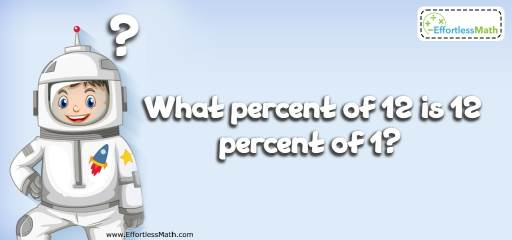# Algebra Puzzle – Critical Thinking 14

This kind of critical thinking challenges can help you develop your creativity. Try using math puzzles to help students develop strategically thinking about math.## Challenge:

What percent of 12 is 12 percent of 1?

### The Absolute Best Book to challenge your Smart Student!

The correct answer is 1 percent.

First, change the word problem into a mathematic equation.
What percent of 12 is 12 percent of 1?
Put variable x for “what percent”. Then,
$$x× 12 = 12\% × 1 ⇒ 12x = 0.12 ⇒ x =\frac{0.12}{12}=0.01=1\%$$

### What people say about "Algebra Puzzle – Critical Thinking 14 - Effortless Math: We Help Students Learn to LOVE Mathematics"?

No one replied yet.

X
30% OFF

Limited time only!

Save Over 30%

SAVE $5 It was$16.99 now it is \$11.99